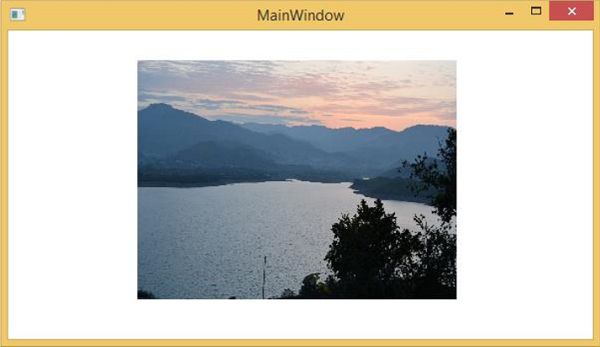# WPF - Multi Touch

Windows 7 and its higher versions have the ability to receive input from multiple touch-sensitive devices. WPF applications can also handle touch input as other input, such as the mouse or keyboard, by raising events when a touch occurs.

WPF exposes two types of events when a touch occurs − touch events and manipulation events. Touch events provide raw data about each finger on a touchscreen and its movement. Manipulation events interpret the input as certain actions. Both types of events are discussed in this section.

The following components are required to develop an application that can respond to touch.

• Microsoft Visual Studio 2010 or later versions.
• Windows 7 or higher version.
• A device, such as a touchscreen, that supports Windows Touch.

The following terms are commonly used when touch input is discussed −

• Touch − Type of user input which can be recognized in Windows 7 or later. Touch input is initiated from a touch-sensitive screen.

• Multi Touch − Type of input which occurs from more than one point simultaneously. In WPF, when touch is discussed, it usually means multi-touch.

• Manipulation − Occurs when touch is inferred as a physical action that is applied to an object. In WPF, manipulation events interpret input as a translation, expansion, or rotation manipulation.

• Touch Device − Represents a device that produces touch input, such as a single finger on a touchscreen.

## Example

To understand all these concepts, let’s create a new WPF project with the name WPFTouchInput.

• Drag a Rectangle from a toolbox to the design window and fill the rectangle with an image or any color. If you want to use an image, then don’t forget to include the image in your solution, otherwise the program will not execute.

• The following XAML code initializes a rectangle with different properties and events.

```<Window x:Class = "WPFMultiTouchInput.MainWindow"
xmlns = "http://schemas.microsoft.com/winfx/2006/xaml/presentation"
xmlns:x = "http://schemas.microsoft.com/winfx/2006/xaml"
xmlns:d = "http://schemas.microsoft.com/expression/blend/2008"
xmlns:mc = "http://schemas.openxmlformats.org/markup-compatibility/2006"
xmlns:local = "clr-namespace:WPFMultiTouchInput"
mc:Ignorable = "d" Title = "MainWindow" Height = "350" Width = "604">

<Window.Resources>
<MatrixTransform x:Key = "InitialMatrixTransform">
<MatrixTransform.Matrix>
<Matrix OffsetX = "200" OffsetY = "200"/>
</MatrixTransform.Matrix>
</MatrixTransform>
</Window.Resources>

<Canvas>
<Rectangle Name = "manRect" Width = "321" Height = "241"
RenderTransform = "{StaticResource InitialMatrixTransform}"
IsManipulationEnabled = "true" Canvas.Left = "-70" Canvas.Top = "-170">
<Rectangle.Fill>
<ImageBrush ImageSource = "Images/DSC_0076.JPG"/>
</Rectangle.Fill>
</Rectangle>
</Canvas>

</Window>
```

Here is the implementation for different manipulation events −

```using System.Windows;
using System.Windows.Input;
using System.Windows.Media;
using System.Windows.Shapes;

namespace WPFMultiTouchInput {

public partial class MainWindow : Window {

public MainWindow() {
InitializeComponent();
}

void Window_ManipulationStarting(object sender, ManipulationStartingEventArgs e) {
e.ManipulationContainer = this;
e.Handled = true;
}

void Window_ManipulationDelta(object sender, ManipulationDeltaEventArgs e) {
Rectangle rectToMove = e.OriginalSource as Rectangle;
Matrix rectsMatrix = ((MatrixTransform)rectToMove.RenderTransform).Matrix;

rectsMatrix.RotateAt(e.DeltaManipulation.Rotation, e.ManipulationOrigin.X, e.ManipulationOrigin.Y);

rectsMatrix.ScaleAt(e.DeltaManipulation.Scale.X, e.DeltaManipulation.Scale.X,
e.ManipulationOrigin.X, e.ManipulationOrigin.Y);

rectsMatrix.Translate(e.DeltaManipulation.Translation.X,
e.DeltaManipulation.Translation.Y);

rectToMove.RenderTransform = new MatrixTransform(rectsMatrix);
Rect containingRect = new Rect(((FrameworkElement)e.ManipulationContainer).RenderSize);

Rect shapeBounds = rectToMove.RenderTransform.TransformBounds(new Rect(rectToMove.RenderSize));

if (e.IsInertial && !containingRect.Contains(shapeBounds)) {
e.Complete();
}

e.Handled = true;
}

void Window_InertiaStarting(object sender, ManipulationInertiaStartingEventArgs e) {
e.TranslationBehavior.DesiredDeceleration = 10.0 * 96.0 / (1000.0 * 1000.0);
e.ExpansionBehavior.DesiredDeceleration = 0.1 * 96 / (1000.0 * 1000.0);
e.RotationBehavior.DesiredDeceleration = 720 / (1000.0 * 1000.0);
e.Handled = true;
}

}
}
```

When you compile and execute the above code, it will produce the following widnow.Now you can rotate, zoom in, zoom out this image with your finger on touch screen.

wpf_input.htm